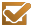Trigonometry, International Edition, 8th Edition - 9780538735490

Trigonometry, International Edition, 8th Edition Larson, Ron Cengage Tecnicos Importados
Avaliações

Por: R\$ 280,96

em até 4x sem juros no cartãoProduto sob Encomenda

Sinopse

This market-leading text continues to provide students and instructors with sound, consistently structured explanations of the mathematical concepts. Designed for a one-term course that prepares students to study calculus, the new Eighth Edition retains the features that have made Trigonometry, International Edition, a complete solution for both students and instructors: interesting applications, cutting-edge design, and innovative technology combined with an abundance of carefully written exercises. Table of Contents P. PREREQUISITES. Review of Real Numbers and Their Properties. Solving Equations. The Cartesian Plane and Graphs of Equations. Linear Equations in Two Variables. Functions. Analyzing Graphs of Functions. A Library of Functions. Transformations of Functions. Combinations of Functions: Composite Functions. Inverse Functions. Chapter Summary. Review Exercises. Chapter Test. Proofs in Mathematics. P.S. Problem Solving. 1. TRIGONOMETRY. Radian and Degree Measure. Trigonometric Functions: The Unit Circle. Right Triangle Trigonometry. Trigonometric Functions of Any Angle. Graphs of Sine and Cosine Functions. Graphs of Other Trigonometric Functions. Inverse Trigonometric Functions. Applications and Models. Chapter Summary. Review Exercises. Chapter Test. Proofs in Mathematics. P.S. Problem Solving. 2. ANALYTIC TRIGONOMETRY. Using Fundamental Identities. Verifying Trigonometric Identities. Solving Trigonometric Equations. Sum and Difference Formulas. Multi

Detalhes do produto

 Peso: 0,4 kg Número de páginas: 624 Ano de edição: 2011 ISBN 10: 053873549X ISBN 13: 9780538735490 Altura: 28 Largura: 21 Comprimento: 3 Assuntos : Ciências Exatas Assuntos : Matemática